#### 3.1 Trigonometric functionsTrigonometric functions are refer to an angle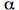and they are divisions of a segment with another one. Therefore the value of a trigonometric function is a real number. We consider a plane cartesian coordinate system, a circumference with radius r, a P point into that circumference and a right triangle (OPP”).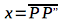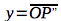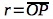• Sine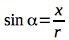• Cosine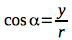• Tangent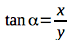• Cotangent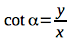There are another two trigonometric functions, but they are used less:

• Secant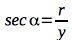Cosecant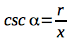In other contexts and subjects (not Topography), the trigonometric functions may appears defined in another way. Remember that we consider positive the clockwise rotation, instead classical mathematics definition for polar coordinate system consider positive the anti-clockwise rotation. The value of trigonometric functions are positive or negative depend the quadrant which the point is located:

 quadrant sine cosine tangent cotangent secant cosecant I + + + + + + II + – – – – + III – – + + – – IV – + – – + –